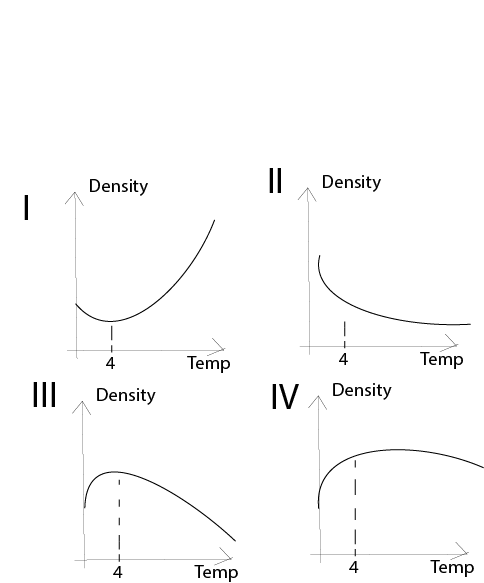## Physics 2018 Past Question | WAEC

Study the following past questions and answers for JAMBWAEC  NECO and Post-JAMB. Get prepared with official past questions and answers for upcoming examinations.

11. An object at rest is said to possess

• A. potential energy
• B. kinetic energy
• C. chemical energy
• D. electrical energy

12. According to Newton’s law of cooling, the heat energy lost per unit area of a body depends on the

I. Surface area

II. Nature of the surface

III. Temperature of the surface

IV. Excess temperature over the surroundings

• A. I and II only
• B. II and IV only
• C. I,II and III
• D. I, II and IV

13.The graph of density against temperature for water is correctly illustrated by

• A. I
• B. II
• C. III
• D. IV

14.  A football is kicked at an angle of 45° to the horizontal over a defense line up with a velocity of 15$$ms^{-1}$$. Calculate the magnitudeof horizontal velocity of the ball at its highest point  [Neglect friction, g = 10$$ms^{-2}$$].

• A. 0.0$$ms^{-1}$$
• B. 5.6$$ms^{-1}$$
• C. 10.6$$ms^{-1}$$
• D. 15.0$$ms^{-1}$$

15. The ice point on the absolute scale of temperature is

• A. 0K
• B. 32K
• C. 100K
• D. 273K

16. Which of the following factors does not increase the rate of evaporation of water in a lake?

• A. Increase in the pressure of the atmosphere
• B. Rise in temperature
• C. Increase in the surface area of the lake
• D. Increase in the speed of the wind

17. The bob of a simple pendulum takes 8.0s to complete 10 oscillations. Determine the frequency of oscillation of the bob.

• A. 0.125Hz
• B. 1.25Hz
• C. 7.50Hz
• D. 8.00Hz

18.The diagram above illustrates the mercury-in-glass thermometer used for determining the temperature of a room. Use the data on it to determine the room temperature.

• A. 3.3°C
• B. 7.0°C
• C. 13°C
• D. 30.0°C

19. Frequency is measured in

• A. meter per second
• B. seconds
• C. hertz

20. Which of the following electromagnetic waves is most energetic?